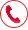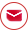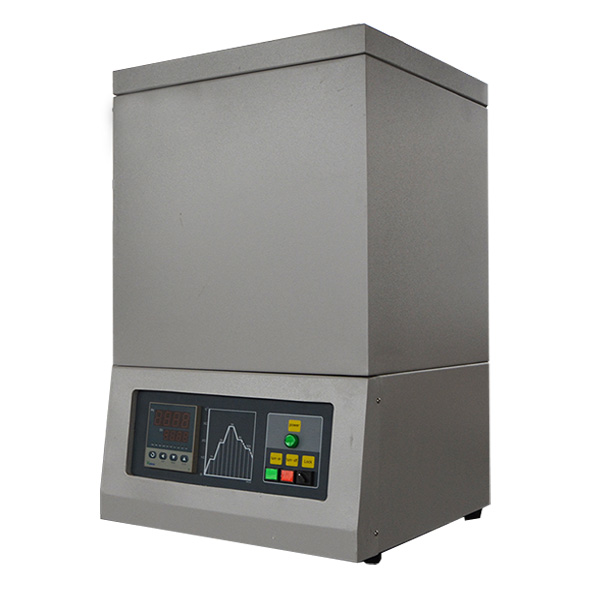+86 18037178440info@lab-furnace.comNEWS

# How should we calculate the power of the high temperature furnace

Time：2020-10-09 14:12
Calculation of the power of the high-temperature furnace: The power must be determined first, while the electric heating efficiency and power factor should be considered. There are two ways to determine the power.One is the heat balance method. According to the law of conservation of energy, the total heat consumed by the resistance high-temperature furnace is equal to the total heat emitted by the electric heating element. The total heat consumed includes the effective heat of heating the metal and the heat loss of the high-temperature furnace. The heat is converted to total power, and the electric heating efficiency is taken into account, and then multiplied by the power reserve coefficient. This coefficient estimates that the productivity of high-temperature furnaces may increase and the heat loss may increase. The power reserve coefficient is for continuous high-temperature furnaces and for intermittent operations. The other type of high temperature furnace is the empirical method, which mainly determines the furnace power according to the furnace volume.

Before calculating the electric heating element, the material of the electric heating element and its connection method should be selected according to the determined power and power supply line voltage.

### HOT PRODUCTS

• Touch screen inclined rotary tubular furnace
• Three phase electric high temperature muffle furnace
• Three temperature zone inclined tube furnace
• Benchtop furnace